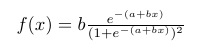# 逻辑回归

LR 是一种简单、高效的常用分类模型——有点奇怪是吧，为什么名字叫做“回归”却是一个分类模型，这个我们稍后再讲。先来看看这个 LR 本身。

LR 的模型函数记作：y=h(x)，具体形式如下：

hθ(x)=11+e−θTx

h(x)=11+e−(a+bx)

h(z)=11+e−z

Sigmoid 这样一个奇怪而别扭的形式到底是谁、因为什么想出来的呢？又怎么想到用它来做分类的呢？

18世纪，随着工业革命的深入；世界经济、科技的发展；美洲的发现，以及随之而来的大移民和北美人口迅猛增长……各个学科对于统计学的工具性需求越来越强烈。

### 指数增长

W(t)表示的是存量，则 W(t) 对 t 求微分的结果 W′(t)就代表了人口/化合物的增长率。

W(t)=aebt

# 逻辑函数

W′(t)=bW(t)−g(W(t))

W′(t)=bW(t)(1–W(t)L)

P(t)=W(t)L

P′(t)=bP(t)(1–P(t))

P(t)=e(a+bt)1+e(a+bt)

P(t)=11+e−(a+bt)

h(x)=11+e−(a+bx)hθ(x)=11+e−θTx

# 追本溯源的理论学习

• 首先，将目标问题定义为一个函数；

• 之后，选取最简单的假设作为其具体形式；

• 然后，用事实数据验证该形式，确认有效后沿用，形成数学模型；

• 一旦当前采用的数学模型出现问题，则对其进行修正（添加修正项），同样借助事实数据来求取修正项的形式，形成新的（升级版）数学模型。

“直接、简单”还比较抽象，但“现有成果”却是看得见摸得着的。

# 线性 VS 非线性

• 线性：二维坐标系中的直线，三维坐标系中的平面……

• 非线性：二位坐标中的曲线（严格的来讲，直线也是一种特殊的曲线，但为了方便而言，我们在此处用“曲”来指代“非直”。“非直”包括“弯曲”，也包括 ReLU 函数这种“一段段拼接的线段”）；三维坐标中的曲面……06-061万+
08-131180
03-12162
03-271769
06-07222
01-25145
09-181万+
07-13458
06-241万+